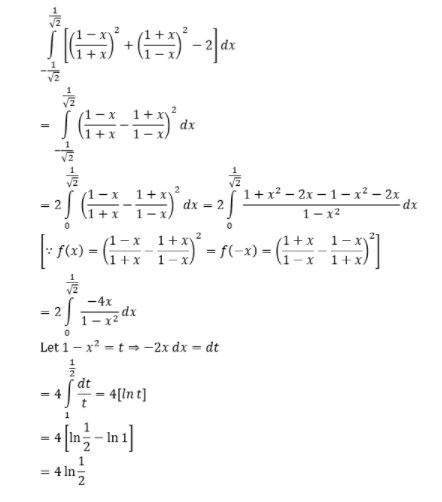# Evaluate $$\int_{\frac{-1}{\sqrt{2}}}^{\frac{1}{\sqrt{2}}}[(\frac{1-x}{1+x})^2 + (\frac{1+x}{1-x})^2 - 2]dx$$

Solution:(0)(0)